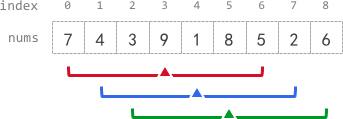### Prefix Sum: Trading Space for Time

This problem can be solved with a Prefix Sum approach. Basically we can calculate and store the partial array sum from 0..i, for each i. That way we can easily compute the average needed (just use long for the prefix sum array since the addition may go past Max.Int). This way we're trading space (O(n)) for time (also O(n)). Code is down below, cheers, ACC.

Medium

You are given a 0-indexed array `nums` of `n` integers, and an integer `k`.

The k-radius average for a subarray of `nums` centered at some index `i` with the radius `k` is the average of all elements in `nums` between the indices `i - k` and `i + k` (inclusive). If there are less than `k` elements before or after the index `i`, then the k-radius average is `-1`.

Build and return an array `avgs` of length `n` where `avgs[i]` is the k-radius average for the subarray centered at index `i`.

The average of `x` elements is the sum of the `x` elements divided by `x`, using integer division. The integer division truncates toward zero, which means losing its fractional part.

• For example, the average of four elements `2``3``1`, and `5` is `(2 + 3 + 1 + 5) / 4 = 11 / 4 = 2.75`, which truncates to `2`.

Example 1:```Input: nums = [7,4,3,9,1,8,5,2,6], k = 3
Output: [-1,-1,-1,5,4,4,-1,-1,-1]
Explanation:
- avg, avg, and avg are -1 because there are less than k elements before each index.
- The sum of the subarray centered at index 3 with radius 3 is: 7 + 4 + 3 + 9 + 1 + 8 + 5 = 37.
Using integer division, avg = 37 / 7 = 5.
- For the subarray centered at index 4, avg = (4 + 3 + 9 + 1 + 8 + 5 + 2) / 7 = 4.
- For the subarray centered at index 5, avg = (3 + 9 + 1 + 8 + 5 + 2 + 6) / 7 = 4.
- avg, avg, and avg are -1 because there are less than k elements after each index.
```

Example 2:

```Input: nums = , k = 0
Output: 
Explanation:
- The sum of the subarray centered at index 0 with radius 0 is: 100000.
avg = 100000 / 1 = 100000.
```

Example 3:

```Input: nums = , k = 100000
Output: [-1]
Explanation:
- avg is -1 because there are less than k elements before and after index 0.
```

Constraints:

• `n == nums.length`
• `1 <= n <= 105`
• `0 <= nums[i], k <= 105`
Accepted
7,404
Submissions
19,468

```public int[] GetAverages(int[] nums, int k)
{
long[] prefixSum = new long[nums.Length];

prefixSum = nums;
for (int i = 1; i < nums.Length; i++)
{
prefixSum[i] = nums[i] + prefixSum[i - 1];
}

int[] retVal = new int[nums.Length];

for (int i = 0; i < retVal.Length; i++)
{
if (i - k >= 0 && i + k < retVal.Length)
{
retVal[i] = (int)((prefixSum[i + k] - prefixSum[i - k] + nums[i - k]) / (2 * k + 1));
}
else
{
retVal[i] = -1;
}
}

return retVal;
}
```